Courses

# RCC Design 3

## 10 Questions MCQ Test RRB JE for Civil Engineering | RCC Design 3

Description
This mock test of RCC Design 3 for Civil Engineering (CE) helps you for every Civil Engineering (CE) entrance exam. This contains 10 Multiple Choice Questions for Civil Engineering (CE) RCC Design 3 (mcq) to study with solutions a complete question bank. The solved questions answers in this RCC Design 3 quiz give you a good mix of easy questions and tough questions. Civil Engineering (CE) students definitely take this RCC Design 3 exercise for a better result in the exam. You can find other RCC Design 3 extra questions, long questions & short questions for Civil Engineering (CE) on EduRev as well by searching above.
QUESTION: 1

### As per IS 456:2000, for the heavily reinforced concrete column, the nominal maximum size of aggregate should be restricted:

Solution:

As per IS 456:2000, Clause 5.3.3.1:

For Heavily reinforced concrete members as in the case of ribs of main beams, the nominal maximum size of aggregate should usually be restricted to 5 mm less than the minimum clear distance between the main bars or 5 mm less than the minimum cover to the reinforcement whichever is smaller.

QUESTION: 2

### A column is held in position and restrained against rotation at one end while not restrained against rotation at other end but held in position. The effective length of such a column as per IS 456 : 2000 is

Solution: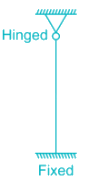Theoretical value = 0.70 L

Recommended value = 0.80 L

QUESTION: 3

### For footing, minimum cover should be

Solution:

As per IS 456 : 2000 Clause number 26.4,

For nominal reinforcement.

For footings, minimum cover shall be 50 mm.

For a longitudinal reinforcing bar in a column nominal cover shall in any case not be less than 40 mm, or less than the diameter of such bar.

In the case of columns of minimum dimension of 200 mm or under, whose reinforcing bars do not exceed 12 mm, a nominal cover of 25 mm may be used.

QUESTION: 4

The reduction coefficient of a reinforced concrete column with an effective length of 4.8 m and size 250 mm × 300 mm is

Solution: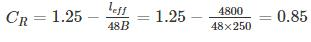QUESTION: 5

The main reinforcement of an RC slab consists of 10 mm bars at 10 cm spacing. If it is desired to replace 10 mm bars by 16 mm bars, then the spacing of 16 mm bars should be

Solution:

The horizontal distance between parallel main reinforcement bars shall not be more than three times the effective depth of solid slab or 300 mm whichever is smaller. The total reinforcement in the slab should remain same. By replacing 10 mm bars by 16 mm bars, the spacing will increase as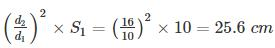QUESTION: 6

An axially loaded column is of 400 mm × 500 mm size. The effective length of the column is 4 m. What is the minimum eccentricity of the axial load for the column along shorter and longer side respectively?

Solution:

As per IS 456:2000, minimum eccentricity equals to unsupported length of column/500 plus lateral dimension/30, subject to minimum of 20 mm.

In this case: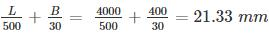along shorter side

And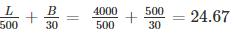mm along longer side.

QUESTION: 7

A plain concrete footing made of M20 concrete is subjected to uniform pressure of 7 Mpa. Its total depth is 700 mm, the permissible maximum projection beyond the face of the column is ________

Solution:

fck = 20 MPa

D = 700 mm

qo = 7 Mpa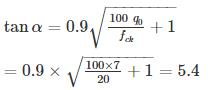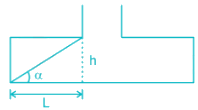∴ tan⁡ α = 700/L ⇒ L = 129.6 ≃ 130mm

QUESTION: 8

An isolated footing of 2 m × 3 m along the plane of bending moment is provided under the column. If the net maximum and minimum pressure on the soil under the footing are 95 kN/m2 and 55 kN/m2 respectively and the axial load is 450 kN, then the bending moment at its base will be (The center of gravity of column and footing coincides)

Solution:

Area of footing = 2 × 3 = 6m2

Section modulus,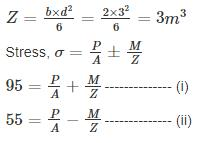From (i) and (ii), we get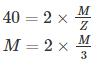⇒ M = 60 kNm

QUESTION: 9

A RC short column of size 460 mm × 460 mm having an effective length of 3.6 m is to be designed using LSM to support an axial service load of 2500 kN

The area of steel in compression is (M20, Fe415)

Solution:

Pu = 1.5 × 2500 = 3750 kN

A = 460 × 460 = 211600 mm2

P= 0.4 fck Ac + 0.67 fy Asc

3750 × 103 = 0.4 × 20 × (211600 - Asc) + 0.67 × 415 Asc

Asc = 7618 mm

QUESTION: 10

A square footing of side 4m is designed to support a square column of 400 mm size. The effective depth of footing is 500 mm. The safe bearing capacity below the footing is 1.5 MPa. The design bending moment for the footing is________

Solution: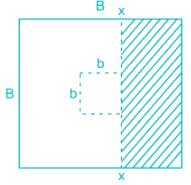The critical section for bending moment is the face of the column:-
Po = Net upward pressure = 1.5 N/mm2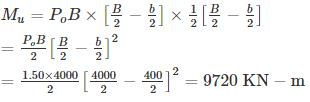• RCC